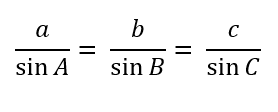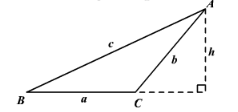# Law of Sines Worksheets

What is the Law of Sines? In the world of trigonometry, there is another law that is used to determine the nature of a triangle, i.e., the law of sines. The law of sines is considered as the relationship between the sides and the oblique angles of the triangles. In other words, it simply states that the ratio of the length of any side of a triangle and the sine of the opposite angle remains the same for all sides of the triangle. In retrospect, for a triangle ABC which is oblique with the sides a, b, and c, the law of sines will be:To use the Law of Sines, you need to know either two angles and one side of the triangle (AAS or ASA) or two sides and an angle opposite one of them (SSA).• ### Basic Lesson

Guides students solving equations that involve an Law of Sines. Demonstrates answer checking. In △ABC, side a = 8, m ∠A = 30° and m ∠C = 55°. Find side c to the nearest tenth of an integer.

• ### Intermediate Lesson

Demonstrates how to solve more difficult problems. Using C = sin-1(.342), we have C = 19.999 = 20° (Since triangle ABC already has an obtuse angle of 110 degrees, we can eliminate the notion that sin is also positioned in Quadrant II, which would give us a second obtuse angle.

• ### Independent Practice 1

A really great activity for allowing students to understand the concept of Law of Sines. Find the length of b to the nearest tenth

• ### Independent Practice 2

Students find the Law of Sines in assorted problems. The answers can be found below.

• ### Independent Practice 3

A really great activity for allowing students to understand the concept of Law of Sines.

• ### Independent Practice 4

Students find the Law of Sines in assorted problems. The answers can be found below.

• ### Homework Worksheet

Students are provided with problems to achieve the concepts of Law of Sines.

• ### Skill Quiz

This tests the students ability to evaluate Law of Sines.